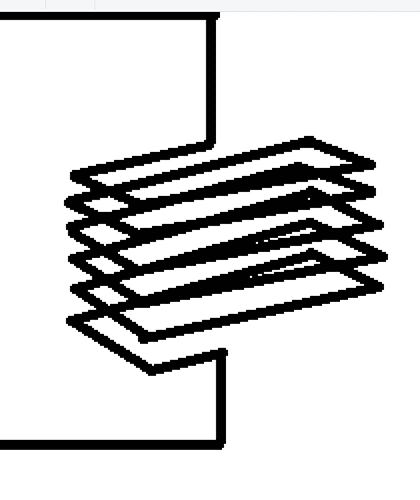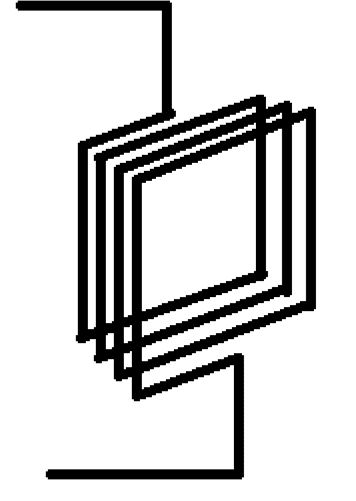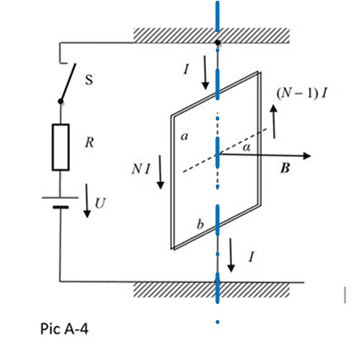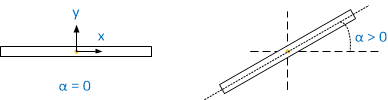# Electromagnetism - movement of a coil in a magnetic field

Ali123
Homework Statement:
Hi, this is not a homework actually, I’m just kind of interested in Physic and I’m solving some more complicated problems. And with this one I’m literally lost. In school we have not been through this and the teacher wasn’t really up to any help. So I would like to ask you for help. Here is the problem:

A rectangular coil with a height a, width b, of copper wire with a diameter of d with a number of N-½ threads (or turns or loops, not sure which is the proper word) (the last thread/loop is only half) is mounted on the vertical conductive and elastic fiber (Pic. A − 4).

Pic A-4 (I'm attaching the picture)
The coil can deflect/deviates about a vertical axis given by the fiber. The coil is located in a homogeneous magnetic field with induction B in the horizontal direction. The coil is connected to a power supply with a voltage U. The current I of the coil is given by the resistance of the R resistor.
When switch S is switched off –( at rest), the plane of the coil with the direction of the magnetic field forms a zero angle α0 (α = 0). If we move the coil from the equilibrium position by a small angle α and release it, it will oscillate around the equilibrium position with the frequency f0.
After switching on the switch, the current I1 starts to go through the electrical circuit.
a) After stabilization of the coil oscillations, the new equilibrium position of the coil is given by the angle α1 of deflection from the initial position. Write the equation for the angle α1
b) After a small deflection from the new equilibrium position and release, the coil starts to oscillate with frequency f1. Determine the frequency f1 as a function of the angle α1.
c) For a given current I1, the angle α1 = fa (B) is a function of the induction B and the frequency f1 = fb (α1) is a function of the angle α1. Plot graphs of both functions for given values.
d) This way can be measured the horizontal component Bh of magnetic field induction. Determine the induction of Bh using the constructed graphs from the measured values I1 = 2.0A, f0 = 10Hz and f1 = 300Hz and the given coil parameters.
Solve the problem in general and then for values: N = 5, a = 10 cm, b = 10 cm, d = 0.20 mm, I1 = 2.0 A
Relevant Equations:
Fm=B*I*l*sinα
f=1/T
v=s/T -->T=s/v
--> f=v/s
So my idea was that to reach the equilibrium position, the final moment of force has to be 0 (so in the end the forces will “eliminate” each other). And I found the equation Fm=B*I*l*sinα, which should characterize the force, which affects wire with the current in a magnetic field, and Fleming’s left-hand rule. But it was all about just one single wire, not the whole coil and it’s kind of difficult for me to imagine how should the rectangular coil move.
I'm attaching 2 pictures (other than the one given) because I'm not sure which way should be the coil oriented#### Attachments

Last edited:

Homework Helper
Hello @Ali123 ,!​

Hi, this is not a homework actually
Doesn't matter: for us it's as homework and will be treated as such. For you it is beneficial to treat it as homework too.

In particular:
Fm=B*I*l*sinα
f=1/T
v=s/T -->T=s/v
--> f=v/s
What are these symbols representing ? (Don't worry, I know --- but I wonder if you know and know where these equations can be applied.)
Tip: don't use both I and l -- it's too easy to mix them up. L is better than l in this case.
And I found the equation Fm=B*I*l*sinα, which should characterize the force, which affects wire with the current in a magnetic field
Look carefully where you found this and maybe you can 'find' something better.
Did you notice your symbol N doesn't appear in any of your equations ? Or ##a## ?
You will also need equations for a so-called torsion balance.
I'm not sure which way should be the coil
I don't understand. Which of your sketches is better, do you think ?

Ali123
Yeah, I didn't mean for you to treat it any special way, it's just that I studied it on my own so I could've missed something or took from wrong sources or using some misleading information.
so the equations: I used the period equation to "complete" the equation of frequency (speed divided by distance, the distance I assume should be calculated from 2πr (since it should be a circular track))
for the second equation, yeah I know the number of loops (N) doesn't appear in it since I found it just as the equation for a single wire, not the whole coil (I tried to find something for the coil, but well was unsuccessful).

To the scatches (sorry I know they aren't the best). So what I was trying to ask by them, was how should the coil be oriented. Normally I would say it would be like thisbut from the picture which was attached to the Problem I wasn't sure whether it's not meant to be oriented like this. I don't know is it better ? Is it understandable what I'm trying to ask?

Thank you I'm going to look for the torsion balance and some equation which will include the rest of the data given, and will see how far I can get.

Homework Helper
it's just that I studied it on my own
Hats off ! On your own it's not easy to get good help when in doubt, even in PF !
the scatches (sorry I know they aren't the best)
Don't apologize: the sketches are perfect for their purpose: to complement and clarify the text. In this case your doubt.

Your second sketch agrees with the picture in the exerciseIf we consider the vertical line through " the vertical conductive and elastic fiber " as the z-axis, and the direction of the external magnetic field ##\vec B\ ## as the x-axis, then the essence of the setup is that the coil is always in a vertical plane and can rotate around the z-axis.

In the description, we read " When switch S is switched off –( at rest), the plane of the coil with the direction of the magnetic field forms a zero angle α0 (α = 0) . "

That means:
the equilibrium position of the coil in the absence of the external B field is in the x-plane.

Then comes a nice bit of almost hidden info: " If we move the coil from the equilibrium position by a small angle α and release it, it will oscillate around the equilibrium position with the frequency f0. "
This has nothing to do yet with electricity or magnetism and everything with elasticity: it's like a mass on a spring that is pulled from the equilibrium position and released.
At small angle ##\alpha > 0## the torque the fibers exert on the coil is proportional to that angle: $$\tau = k\alpha$$ This ##k## is the equivalent of the spring constant ##k##.​
Torque ##\tau## is pointing in the negative z-direction as can be seen from a top view:​A clockwise torque is needed to restore ##\alpha## to zero. In other "words":​
$$\tau_z = -k\alpha$$​
And the torque is proportional to the angular acceleration​
$$\tau = I\ddot\alpha$$(we can't use the habitual symbol for angular acceleration, since we already use it for the angle ##\alpha##. With the dot we designate a time derivative, as in: ##\omega = \dot\alpha## and angular acceleration is ##\dot \omega = \ddot\alpha##).​
I is the moment of inertia -- also confusing when there's current around, but geez...​
(and we don't know this moment of inertia yet)​
So we have$$\tau_z = I \ddot\alpha = -k\alpha$$which leads to harmonic rotary oscillations with frequency ##\displaystyle{f_0 = {1\over 2\pi}\sqrt{k\over I}}## (all familiar and clear ?)​
(##I## and also ##k## unknown thus far)​
wow.​

Then things start to happen:
After switching on the switch, the current I1 starts to go through the electrical circuit.
a) After stabilization of the coil oscillations, the new equilibrium position of the coil is given by the angle α1 of deflection from the initial position. Write the equation for the angle α1

Two ways to deal with this: fundamental and pragmatic. Your Fm=B*I*l*sinα is in between.
My preference is the fundamental path using the Lorentz force.
Best if you google force on a current-carrying loop in a magnetic field and pick one you like.
See if you can find the Fm=B*I*l*sinα . (Note: don't get distracted by the NI and the (N-1)I. Assume N >> 1 and use N-½ for both).

Basically the coil becomes an electromagnet and wants to align with the external field. a lot of work ahead !

By the way, did you find this in a lab instruction or is it a genuine 'dry' textbook exercise ?

(Long post ! running out of time)

##\ ##

•docnet
Ali123

More of a dry textbook exercise, found it on a page in my mother language, could be translated to something like interesting complex physics problems. But there were even some lab experiments.

•BvU
•